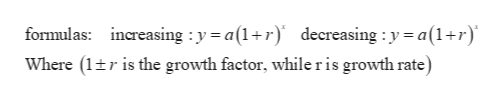# Given the following exponential growth function, identify the growth rate in percentage form:Q=10,000⋅3.0Tr=

Question
19 views

Given the following exponential growth function, identify the growth rate in percentage form:

Q=10,000⋅3.0T

r=

check_circle

Step 1

Here the given exponential growth function is Q=10,000*(3)T.

When the growth factor is greater than 1 then the exponential form repr...help_outlineImage Transcriptioncloseformulas: ncreasing y a(1+r) decreasing : y = a(1+r) Where (1tris the growth factor, while ris growth rate) fullscreen

### Want to see the full answer?

See Solution

#### Want to see this answer and more?

Solutions are written by subject experts who are available 24/7. Questions are typically answered within 1 hour.*

See Solution
*Response times may vary by subject and question.
Tagged in

### Algebra